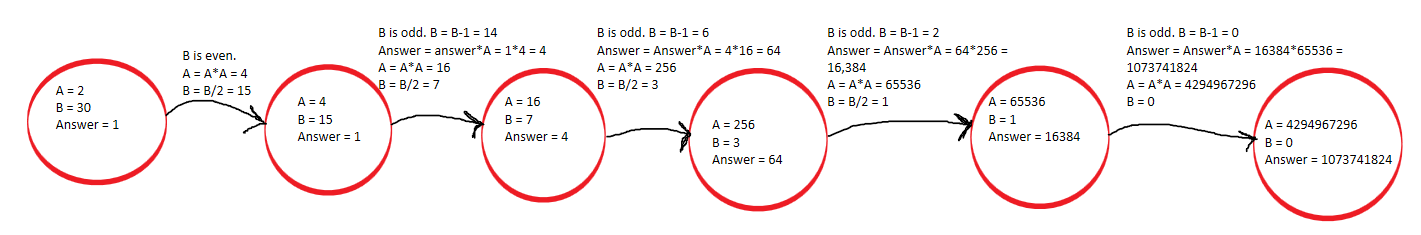New update is available. Click here to update.

# Binary Exponentiation

## Introduction

Exponentiation is a mathematical operation written as ab, which means the value when a is multiplied by itself b times. Here, a is called the base, and b is called the exponent or the power.

## Problem Statement

Given a number a and another number b, compute a^b.

For example,

Input

``a  = 2, b = 14``

Output

``16384``

Explanation: Multiplying 2 to itself 14 times gives 16384.

## Solution Approach

### Approach 1: Brute Force

The most straightforward idea is to go by the definition of exponential and multiply a to itself b times. We run a loop for b times and keep multiplying a to answer. Also, the answer should be initiated by 1.

#### Pseudocode:

``````function exponentiation(int a, int b){
for(int i=0;i<b;i++){
}
}``````

#### C++ implementation

``````#include<bits/stdc++.h>
using namespace std;

int exponentiation(int a, int b){
for(int i=0;i<b;i++){
}
}

int main()
{
int a = 2;
int b = 14;
cout<<exponentiation(a,b)<<endl;
}``````

#### Java Implementation

``````public class Main
{
public static int exponentiation(int a, int b){
for(int i=0;i<b;i++){
}
}
public static void main(String[] args) {
int a = 2;
int b = 14;
System.out.println(exponentiation(a,b));
}
}
``````

#### Python Implementation

``````def exponentiation(a, b):
for i in range(b):

a = 2
b = 14
print(exponentiation(a, b))``````

Output

``16384``

#### Complexity

Time complexity

O(b), where b is the exponent

Reason: Since we’re multiplying a to the answer b times using a loop, the time complexity will be O(b).

Space complexity

O(1)

Reason: All the spaces taken are constant.

### Approach 2: Binary exponentiation

This is the most efficient approach to do exponentiation. We need to calculate ab, which can also be written as (a2)b/2. Notice that computing a2 takes only constant time, and the whole computation steps are reduced to b/2 steps from b steps. Thus, now we need to calculate xb/2, where x  = a2. But, notice that if b is odd, then b/2 will be a decimal, and calculating that is not easy. Also, if b is odd, we can make it even. How? So, xb can be written as x*(xb-1). Thus, whenever we encounter an odd b, multiply x to the answer and reduce the value of b by 1. Then, keep dividing the power by 2 as long as it is even, and keep replacing the base by its square.

Let’s analyze the difference between the above two approaches for an example.

Assume a = 2, b = 30.• The number of steps taken by approach 1 is 30.
• The number of steps taken by approach 2 is 4, equal to log2(30).

Thus, we can see that the second approach is very efficient.

#### Pseudo - code:

``````function exponentiation(int a, int b){
while(b>0){
if(b%2==1){
b--;
}
a = a*a;
b = b/2;
}
}``````

#### C++ implementation

``````#include<bits/stdc++.h>
using namespace std;

int exponentiation(int a, int b){
while(b>0){
if(b%2==1){
b--;
}
a = a*a;
b = b/2;
}
}

int main()
{
int a = 2;
int b = 14;
cout<<exponentiation(a,b)<<endl;
}``````

#### Java Implementation

``````public class Main
{
public static int exponentiation(int a, int b){
while(b>0){
if(b%2==1){
b--;
}
a = a*a;
b = b/2;
}
}
public static void main(String[] args) {
int a = 2;
int b = 14;
System.out.println(exponentiation(a,b));
}
}
``````

#### Python Implementation

``````def exponentiation(a, b):
while b > 0:
if b%2==1:
b -= 1

a *= a
b //= 2

a = 2
b = 14
print(exponentiation(a, b))``````

Output

``16384``

#### Complexities

Time complexity

O(log2b), where b is the exponent

Reason: Since we’re dividing b by 2 in each step, the total number of steps will be log2b.

#### Space complexity

O(1)

Reason: All the spaces taken are constant.

Must read decimal to binary c++

1. What is exponentiation?
Exponentiation is a mathematical operation written as ab, which means the value a is multiplied by itself b times.

2. What is the time complexity of the binary exponentiation method?
The time complexity of the binary exponentiation method is log(b), where b is the exponent.

3. Can exponentiation be done in O(logn) time?
Yes, exponentiation can be done in O(logn) time.

4. What should we do if an exponentiation's value is too large to fit in the integer range?
If any answer's value is so large that it can’t fit in the integer range, take its modulo with a prime number. Generally, that prime number is 10^9 + 7.

5. Where can binary exponentiation be used?
Binary exponentiation can efficiently compute Fibonacci numbers and apply a permutation on an array k times.

## Key Takeaways

This article discussed the method of doing binary exponentiation. These mathematical concepts are used to make our solutions faster. You can read and learn more mathematical concepts here

Are you planning to ace the interviews of reputed product-based companies like Amazon, Google, Microsoft, and more?

Attempt our Online Mock Test Series on CodeStudio now!

Happy Coding!